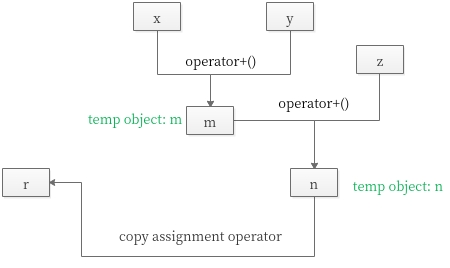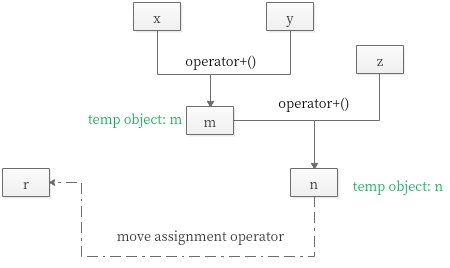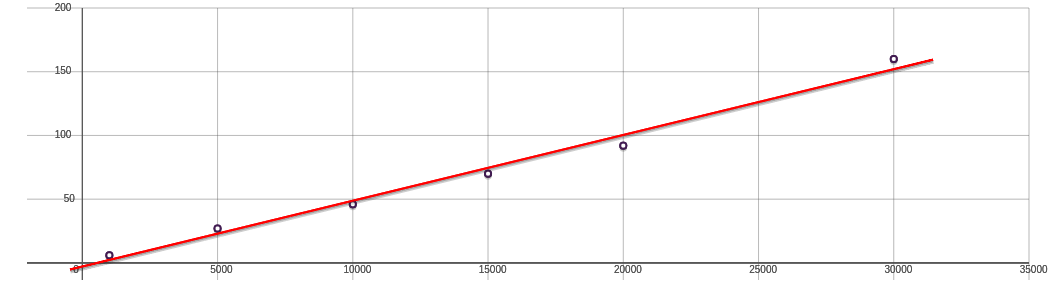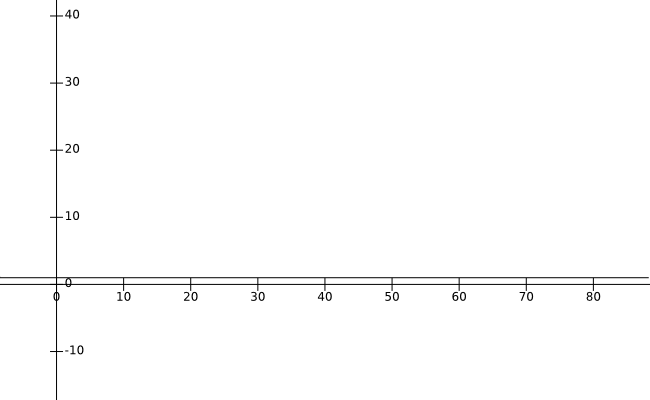Many C++ beginners struggling with “move” semantics since C++11. Fortunately, there are a collection of online materials on move semantics (See: Recommended materials). However, few articles mention why we need move semantics and how it can significantly increase the performance of your applications. Let’s begin this journey directly.

## A Vector class without move semantics

Here, We can define a variable of our new type `Vector`. Note that my Vector not the one from the standard library! We call class Vector a container of `double`s.

``````class Vector {
private:
double* elem; // elem points to an array of sz doubles
int sz;
public:
Vector(int s);
~Vector() { delete[] elem; }

Vector(const Vector& a);               // copy constructor
Vector& operator=(const Vector& a);    // copy assignment

double& operator[](int i);
const double& operator[](int i) const;

int size() const;
};
``````

We need to define copy semantics. Copying of an object of a class is defined by two members: `a copy constructor `and `a copy assignment`.

Copy constructor:

``````Vector::Vector(const Vector& a)   // copy constructor
:elem{new double[a.sz]},     // allocate space for elements
sz{a.sz}
{
for (int i=0; i!=sz; ++i)    // copy elements
elem[i] = a.elem[i];
}
``````

Copy assignment:

``````Vector& Vector::operator=(const Vector& a)     // copy assignment
{
double* p = new double[a.sz];
for (int i=0; i!=a.sz; ++i)
p[i] = a.elem[i];
delete[] elem;         // delete old elements
elem = p;
sz = a.sz;
return *this;
}
``````

Consider:

``````Vector operator+(const Vector& a, const Vector& b)
{
if (a.size()!=b.size())
throw Vector_size_mismatch{};

Vector res(a.size());  // Note: construct an temporary object
for (int i=0; i!=a.size(); ++i)
res[i]=a[i]+b[i];
return res;
}
``````

Returning from a + involves copying the result out of the local variable res and into some place where the caller can access it. We might use this + like this:

``````void f(const Vector& x, const Vector& y, const Vector& z)
{
Vector r;
// ...
r = x+y+z;
// ...
}
``````

That would be copying a Vector at least twice (one for each use of the + operator). The figure below illustrates what is going on “under the hood” of the `r = x+y+z; `:Figure 1. Copy assignment

The most embarrassing part is that `temp object n` in operator+() is never used again after the copy. We didn’t really want a copy; we just wanted to get the result out of a function: we wanted to move a Vector rather than copy it.

## A Vector class with move semantics

we wanted to move a Vector rather than copy it. Fortunately, we can state that intent:

``````class Vector {
// ...

Vector(const Vector& a);               // copy constructor
Vector& operator=(const Vector& a);    // copy assignment

Vector(Vector&& a);                    // move constructor
Vector& operator=(Vector&& a);         // move assignment
};
``````

As is typical, Vector’s move constructor is trivial to define:

``````Vector::Vector(Vector&& a)
:elem{a.elem},          // "grab the elements" from a
sz{a.sz}
{
a.elem = nullptr;       // now a has no elements
a.sz = 0;
}
``````

Of course, we need a move assignment in addition to the move constructor:

``````Vector& Vector::operator=(Vector&& a)     // move assignment operator
{
delete[] elem;         // delete old elements
elem = a.elem ;
sz = a.sz;

a.elem = nullptr;       // now a has no elements
a.sz = 0;
return *this;
}
``````

The compiler will choose the move constructor to implement the transfer of the return value out of the function. This means that `r=x+y+z`will involve no copying of Vectors. Instead, Vectors are just moved.`r=x+y+z`can now be presented as:Figure 2. Move assignment

Instead of an expensive copy operation you can use a cheap move operation. I will compare in this post the performance of the copy and move semantic for the containers `Vector`.

## The performance differences

I use different size of doubles(such as 1000,10000, 100000..) to measure the CPU cycles of `copy assignment` and `move assginment` respectively. Figure 3 and Figure 4 show a plot of the number of clock cycles required by the two functions for a range values of `Vector r`.

copy assignment:Figure 3. Performance of the copy assingnment. The slope of the lines indicates the number of clock cycles per elements. (CPE)

move assginment:Figure 4. Performance of the move assingnment. No matter how many elements of the Vector, CPU cycles can by approximated by the equations: cycles = 1,

This’s why we love move semantics: less memory usage and fewer copy operations，thereby leading to less CPU cycles.

#### My benchmark code example:

Gist: cpp_move_semantics.cpp

#### Code snippet reference:

A Tour of C++, Second Edition, Bjarne Stroustrup